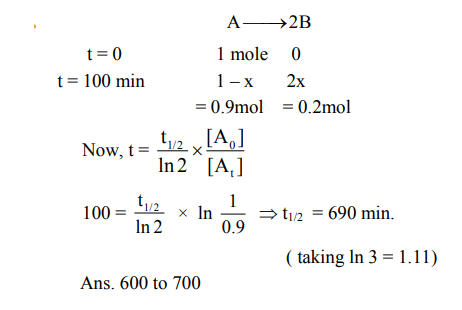# For the first order reactionQuestion:

For the first order reaction $\mathrm{A} \rightarrow 2 \mathrm{~B}, 1$ mole of reactant A gives $0.2$ moles of B after 100 minutes. The half life of the reaction is ...... min. (Round off to the nearest integer).

$[$ Use $: \ln 2=0.69, \ln 10=2.3$

Properties of logarithms : $\ln x^{y}=y \ln x$;

$\left.\ln \left(\frac{x}{y}\right)=\ln x-\ln y\right]$

(Round off to the nearest integer)

Solution: